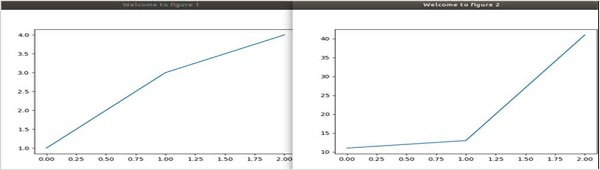# How to show two figures using Matplotlib?

We can use the method, plt.figure(), to create the figures, and then, set their titles by passing strings as arguments.

## Steps

• Create a new figure, or activate an existing figure, with the window title “Welcome to figure 1”.

• Draw a line using plot() method, over the current figure.

• Create a new figure, or activate an existing figure, with the window title “Welcome to figure 2”.

• Draw a line using plot() method, over the current figure.

• Using plt.show(), show the figures.

## Example

from matplotlib import pyplot as plt

plt.figure("Welcome to figure 1")
plt.plot([1, 3, 4])
plt.figure("Welcome to figure 2")
plt.plot([11, 13, 41])

plt.show()

## Output